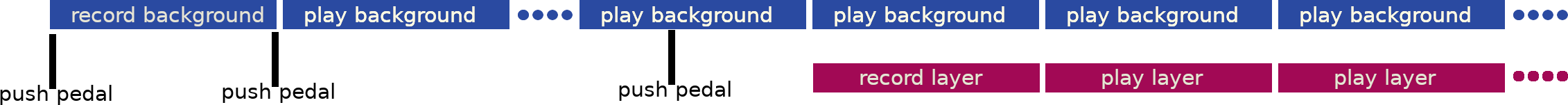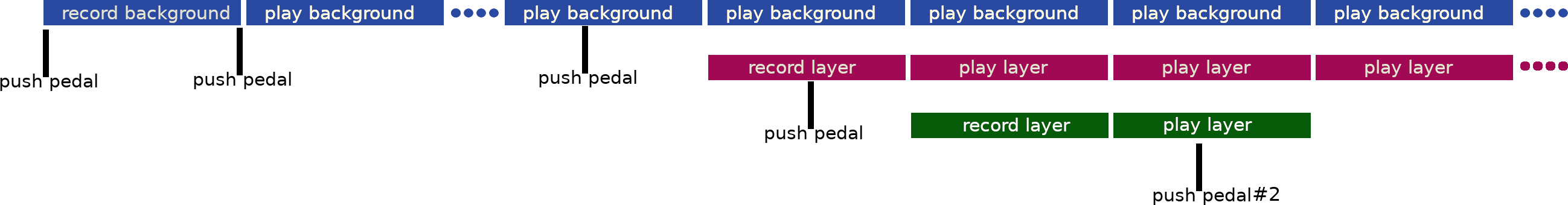# Creating a looper in shell part 3: multiple layers

To understand this article better it is advised to have read the previous one.

In the previous article, we saw how to add a layer to the loop, here was the script behavior:This aims at adding multiple layers by pressing any key, and being able to remove last layer by pressing `a` (pedal #2).# programming pedals

As mentionned earlier, I’m using cheap pedals.

I’m using footswitch to program pedals.

For example, here is how to get one of them to simulate a `a` keypress:

``````\$ sudo ./footswitch -k a
``````

I’ll be using two pedals, one for adding a layer, one for removing last layer.

# Let’s add layers

Here are diffs between last version and new one (in red are the old lines and in green the new ones).

Let’s modify the input function so that it returns `dd` return code and so that it sets a variable (`key` with the key that was pressed):

` input() {`
`   stty raw`
`+  key=\$(dd bs=1 count=1 2> /dev/null)`
`+  rc=\$?`
`+  [ \$rc -ne 0 ] && return \$rc`
`   stty -raw`
`+  return 0`
` }`

Instead of playing one layer (`layer.wav`), we play a bunch of them (`layer1.wav`, `layer2.wav`, …) based on `\$layer_number` variable. Instead of returning one pid, we return a list op PIDs.

` input() {`
`+play_layers() {`
`+  play_layer_pids=""`
`+  for i in \$(seq \$layer_number)`
`+  do`
`+    aplay layer\$i.wav &`
`+    play_layer_pids="\$! \$play_layer_pids"`
`+  done`
` }`

We change current layer to record name to `layer\${layer_number}.wav` instead of `layer.wav` (after incrementing it):

` record_layer() {`
`   record_layer_pid=""`
`   if \$(cat should_record_layer)`
`   then`
`+    layer_number=\$[ \$layer_number + 1 ]`
`+    arecord -f S16_LE -r 48000 -D hw:1,0 layer\${layer_number}.wav &`
`     record_layer_pid=\$!`
`     echo false > should_record_layer`
`   fi`
` }`

We add a function to remove the last layer registered if `should_remove_layer` file contains `true`, by decrementing `\$layer_number`:

`+remove_layer() {`
`+  record_layer_pid=""`
`+  if \$(cat should_remove_layer)`
`+  then`
`+    layer_number=\$[ \$layer_number - 1 ]`
`+    echo false > should_remove_layer`
`+  fi`
`+}`
`+`

We initialize `should_remove_layer` to false, layer_number to 0, and rename `play_layer` to `play_layers` and `play_layer_pid` to `play_layer_pids`:

` main_loop() {`
`   echo false > should_record_layer`
`+  echo false > should_remove_layer`
`+  layer_number=0`
`   while true`
`   do`
`+    remove_layer`
`+    play_layers`
`+    record_layer`
`     aplay background.wav`
`     [ \$? -ne 0 ] && break`
`+    [ -n "\$record_layer_pid" ] && kill \$record_layer_pid`
`+    [ -n "\$play_layer_pids" ] && kill \$play_layer_pids`
`   done`
` }`

Instead of waiting for only one input (since there was only one layer), we wait for multiple input in a loop. If “a” is pressed, we notify that we `should_remove_layer`. Overwise, we notify that we `should_record_layer`:

`+input_loop() {`
`+  while true`
`+  do`
`+    input`
`+    [ "\$key" = \$'\003' ] && break`
`+    if [ "\$key" = "a" ]`
`+    then`
`+      echo true > should_remove_layer`
`+    else`
`+      echo true > should_record_layer`
`+    fi`
`+    sleep 1`
`+  done`
`+}`
`+`
` record_background`
` main_loop &`
` main_loop_pid=\$!`
`+input_loop`
` wait \$main_loop_pid`

# The whole script

``````#!/usr/bin/env sh

input() {
stty raw
key=\$(dd bs=1 count=1 2> /dev/null)
rc=\$?
[ \$rc -ne 0 ] && return \$rc
stty -raw
return 0
}

record_background() {
input
arecord -f S16_LE -r 48000 -D hw:1,0 background.wav &
pid=\$!
sleep 1
input
kill \$pid
}

play_layers() {
play_layer_pids=""
for i in \$(seq \$layer_number)
do
aplay layer\$i.wav &
play_layer_pids="\$! \$play_layer_pids"
done
}

record_layer() {
record_layer_pid=""
if \$(cat should_record_layer)
then
layer_number=\$[ \$layer_number + 1 ]
arecord -f S16_LE -r 48000 -D hw:1,0 layer\${layer_number}.wav &
record_layer_pid=\$!
echo false > should_record_layer
fi
}

remove_layer() {
record_layer_pid=""
if \$(cat should_remove_layer)
then
layer_number=\$[ \$layer_number - 1 ]
echo false > should_remove_layer
fi
}

main_loop() {
echo false > should_record_layer
echo false > should_remove_layer
layer_number=0
while true
do
remove_layer
play_layers
record_layer
aplay background.wav
[ \$? -ne 0 ] && break
[ -n "\$record_layer_pid" ] && kill \$record_layer_pid
[ -n "\$play_layer_pids" ] && kill \$play_layer_pids
done
}

input_loop() {
while true
do
input
[ "\$key" = \$'\003' ] && break
if [ "\$key" = "a" ]
then
echo true > should_remove_layer
else
echo true > should_record_layer
fi
sleep 1
done
}

record_background
main_loop &
main_loop_pid=\$!
input_loop
wait \$main_loop_pid
``````

# Conclusion

The whole script is now available in its own repo. Of course, patches are welcome !

I use this script on a daily basis, and there are tons of things that could be polished. Maybe I’ll rewrite it in some other language :)